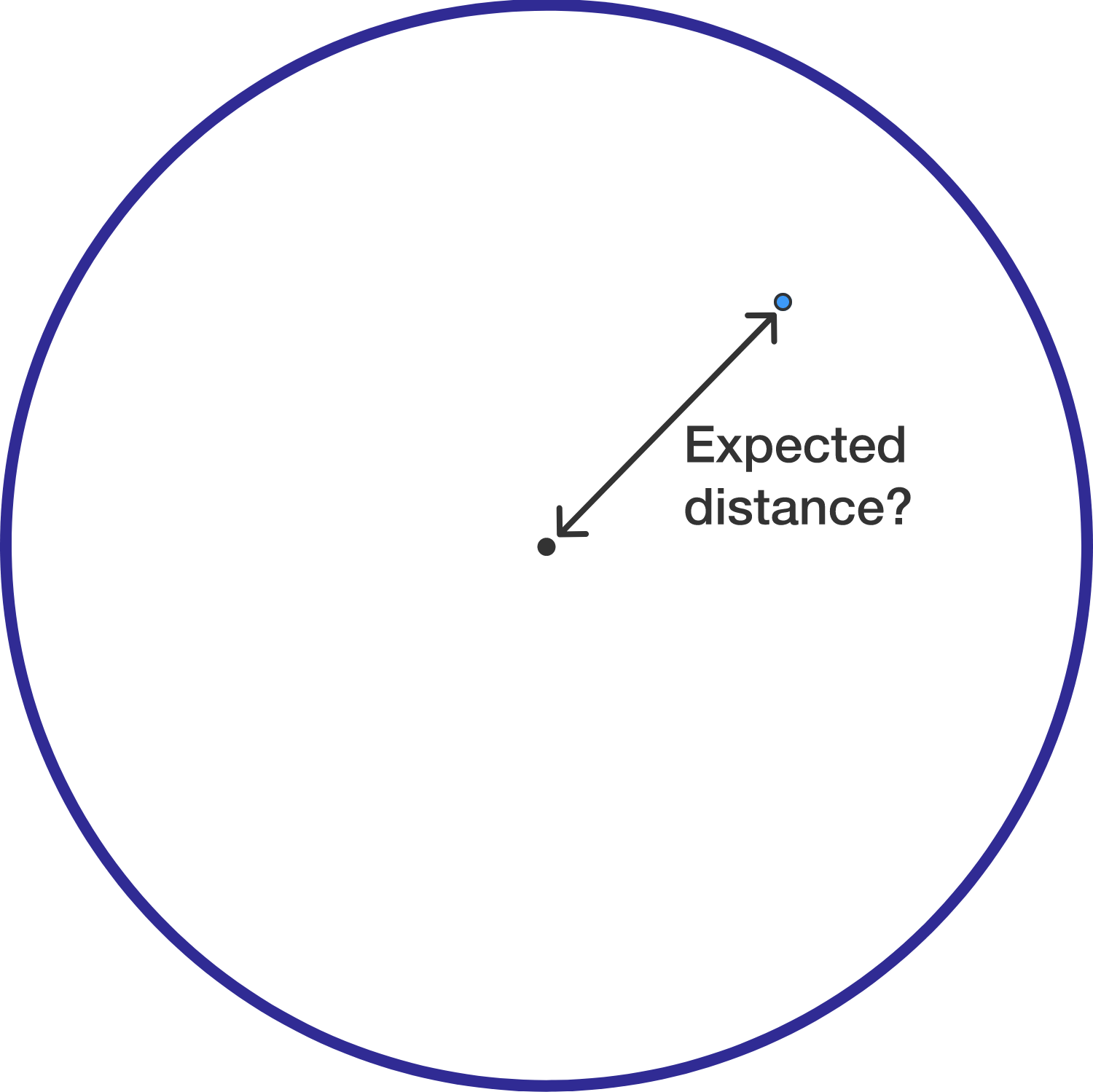# Expected Distance Of Two Points In A Circle

Probability Level 2A point is chosen randomly (by distribution of area) on the inside of a unit circle. Find the expected value of its distance from the center of the circle.

If you get your answer as $\dfrac{a}{b}$, where $a$ and $b$ are coprime positive integers, submit your answer as $a+b$.

×

Problem Loading...

Note Loading...

Set Loading...